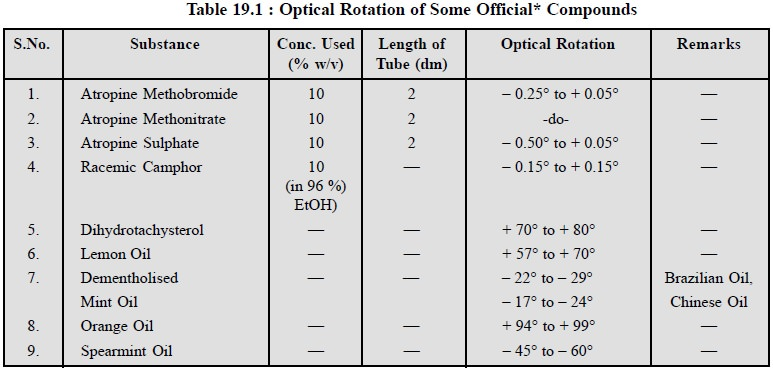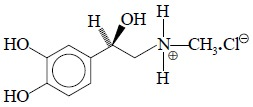Home | | Pharmaceutical Drug Analysis | Determination of Optical Activity of Pharmaceutical Substances

# Determination of Optical Activity of Pharmaceutical Substances

The two characteristic parameters related to optical activity of the pharmaceutical substances, namely : (a) optical rotation, and (b) specific optical rotation, can be measured satisfactorily by the help of a Polarimeter as stated below :

DETERMINATION OF OPTICAL ACTIVITY OF PHARMACEUTICAL SUBSTANCES

The two characteristic parameters related to optical activity of the pharmaceutical substances, namely : (a) optical rotation, and (b) specific optical rotation, can be measured satisfactorily by the help of a Polarimeter as stated below :

## 1. DETERMINATION OF OPTICAL ROTATION OF PHARMACEUTICAL SUBSTANCES

The optical rotation of a number of pure pharmaceutical substances may be measured accurately by noting the angle through which the plane of polarization is rotated when polarized light passes through the substance, if liquid or through a solution of the substance, if solid.

A few typical examples of ibuprofen and levodopa are discussed below :

### 1.1. Ibuprofen

Materials Required : 2.5% (w/v) solution of ibuprofen and a polarimeter ;

Procedure : First and foremost it is absolutely necessary to check the linearity of the scale of a polarimeter either using certified quartz plates or using known solution of sucrose.

The sample tube of the polarimeter is rinsed with the drug solution (2.5% w/v) and filled up with the same solution. The end glass-windows are closed properly. The angle of rotation of ibuprofen is now meas-ured at 19.5° to 20.5°, using the D-line of polarized sodium light. Take at least five measurements and determine the mean value.

### 1.2. Levodopa

Theory : It has been observed that the specific rotation of levodopa in the visible region is rather on the lower side i.e., ([α]20D = – 12° in 1 M hydrochloric acid). Therefore, it is necessary to enhance the optical rotation to a reasonable extent by some suitable means. It is, however, achieved by the formation of a com-plex with hexamine.

Materials Required : Dried levodopa : 0.2 g ; hexamine : 5.0 g ; hydrochloric acid (1 M) : 50 ml (dissolve 85 ml of HCl in 1 L of DW) ;

Procedure : Dissolve a quantity equivalent of 0.2 g of the dried substance and 5 g of hexamine in 10 ml of 1 M hydrochloric acid, add sufficient 1 M HCl to produce 25 ml and allow to stand for 3 hours, protected from light. The optical rotation is measured by a previously calibrated polarimeter.

Optical Rotation : – 1.27° to – 1.34°

### 1.3 Cognate Assays

The optical rotation of a number of substances official in the pharmacopoeia may be determined con-veniently as stated in Table 19.1.## 2. DETERMINATION OF SPECIFIC OPTICAL ROTATION OF PHARMACEUTICAL SUB-STANCES

The specific optical rotation of a solid substance is usually determined by measuring the angle of rotation at the wavelength of the sodium D-line at a temperature of 20°C, and calculating the result with reference to a layer 1 dm thick of a solution containing 1 g of the substance per ml. It is pertinent to mention here that the specific optical rotation of a solid is always expressed to a given solvent and concentration.

Theory : As pure adrenaline is sparingly soluble in distilled water, therefore, its solution is made in 1 M hydrochloric acid whereby the N-atom gets protonated and results into the formation of a quaternary ammonium compounds as shown in under :Materials Required : 4% w/v solution of adrenaline in 1 M HCl (dissolve 85.0 ml of HCl in 1 L of DW) ;

Procedure : Determine the angle of rotation of the freshly prepared 4% w/v solution of adrenaline in 1 M hydrochloric acid with the help of a previously checked polarimeter. The mean value of at least five similar determinations is employed in the calculation of the specific optical rotation.

Calculations : Calculate the specific optical rotation using the following expression, namely :where, l = the length in dm of the polarimeter tube,

d = the relative density of the substance, and

c = the concentration of the substance expressed as a percentage w/v.

### 2.2. Cognate Assays

The specific optical rotation of a large number of potent pharmaceutical substances may be determined by the above mentioned procedure but specific concentrations and method of preparation of solutions is accord-ing to the official compendium as stated in Table 19.2 below :Study Material, Lecturing Notes, Assignment, Reference, Wiki description explanation, brief detail
Pharmaceutical Drug Analysis: Polarimetry : Determination of Optical Activity of Pharmaceutical Substances |